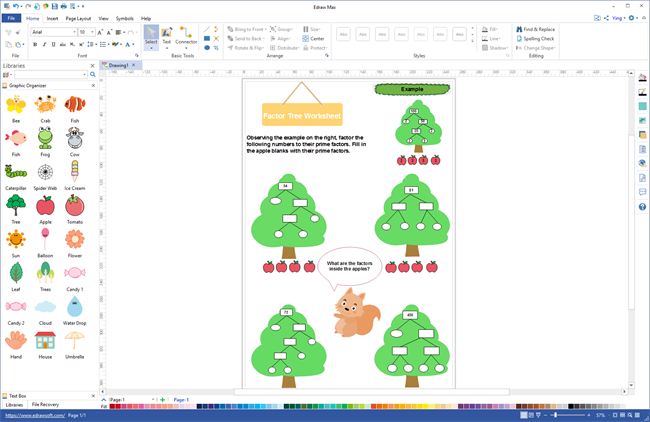# Free Factor Tree Worksheet Templates

> Free Factor Tree Worksheet Templates
Posted by Allison Lynch |
Factor tree is a special diagram to find all of the prime number factors of a number, which is commonly used for primary school study. Below are editable factor tree worksheet templates for free download. Get lovely factor tree worksheet templates for your students.

A factor tree serves as a visualized aid to make the prime factorization process go smoothly. Factor tree worksheets are useful tools to track all prime factors that a number contains. Using a factor tree worksheet in math class will save the teacher much time and attract students to do the work gladly. Students nowadays who are facing massive homework are prone to use factor tree worksheet rather than traditional long texts with poor formats.

### Free Factor Tree Worksheet Templates

The following are simple factor tree worksheets created by Edraw graphic organizer maker. You can choose the template you prefer to customize for your needs. The factor tree worksheets displayed below are free to download, convenient to customize and flexible to use. Click on the picture to download them for free.

#### Factor Tree Worksheet Templates - Cartoon

The following is a cartoon factor tree worksheet. The factor tree is placed inside a green tree with a trunk below which are apples that students can fill in with prime factors. This kind of factor tree work sheet suits primary students who are addicted to cartoon best.

#### Factor Tree Worksheet Templates - Sketch

Different from the cartoon factor tree worksheet displayed above, the one below is less colorful and vivid but more concise and easy to make. Below the factor tree, students need to complete the equation with the prime factors.

### Edit Templates with Factor Worksheet Maker

Do the templates above attract you? Do you want to create factor tree worksheets within minutes? Download Edraw factor tree worksheet maker and edit factor tree worksheet templates above! They are editable vector files. Replace the contents with your own and make them your factor tree worksheets in minutes.Try this easy factor tree worksheet maker:

#### Related Articles: